# How to analyze data using SPSS ( part-5) how to merge file in SPSS?

Merge or combing two data set(add case):

What do we mean by add case?

Add case means combing or merge two data set where the variables of the two data set are same and their name also.
suppose we have a data set named "data set1" where the variables are "id","gender","age","gpa".
and there exist data of 10 students.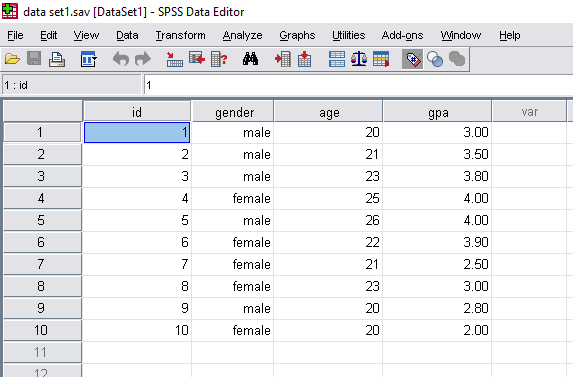Data set 1

Again we have 2nd data set named "data set2" where the variables are same as "data set1"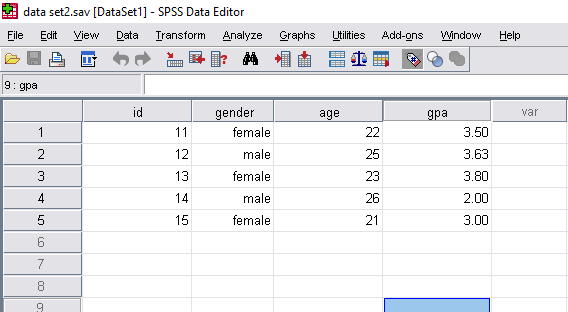Data set 2

Now we have to combine two data set.

• At first we have to go to menu bar and select
Then there open a new box named "Add cases to data set1" and we have to select "An external spss data file" and click on "Browse" and select the second data set which we want to combine with "data set1"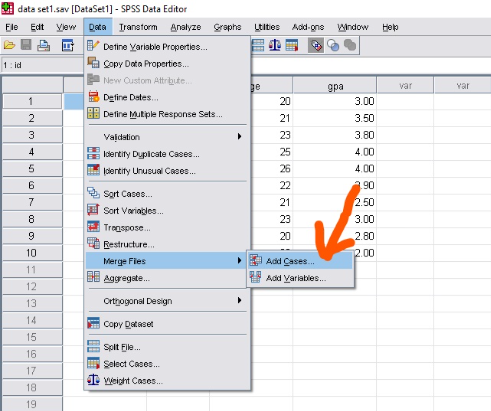Adding cases

↓Adding cases

After selecting the target file we have to click on "continue" and there open a new box . Now we have to click on "ok"
Finally our two data set are combined as below:New data set with all cases

Merge or combining two data set(Add variables):

What do we mean by add variables?
-Add variables means merge or combing two data set where the variables are totally different from each other data set. we have to combine the variables of two data set.

Note: If there exist same variables in two data , one will be excluded after merge.
And also, the length of the data or size of the data in two data set must be same.

suppose we have a data set named "data set1" where the variables are "id","gender","age","gpa".

and there exist data of 10 students.Data set 1

Again we have 2nd data set named "data set2" where the variables are "id", "family_member" and there exist data of 10 students.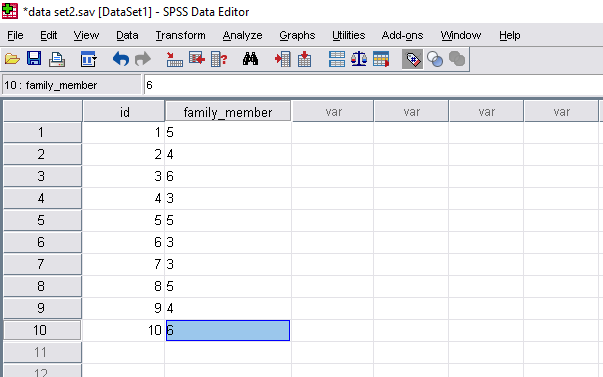Data set 2

Now we have to combine two data set.

• At first we have to go to menu bar and select
Then there open a new box named "Add variables to data set1" and we have to select "An external spss data file" and click on "Browse" and select the second data set which we want to combine with "data set1"Adding valuesAdding values

After selecting the target file we have to click on "continue" and there open a new box . Now we have to click on "ok" and if there exist same variable in the data sets they are excluded automatically .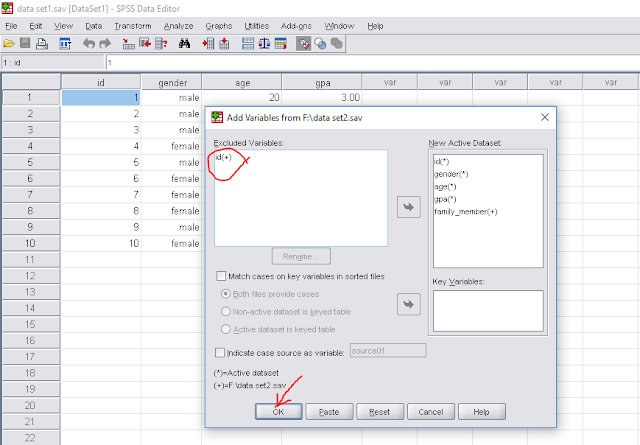Include/exclude variable

And finally we get the combine data set as below: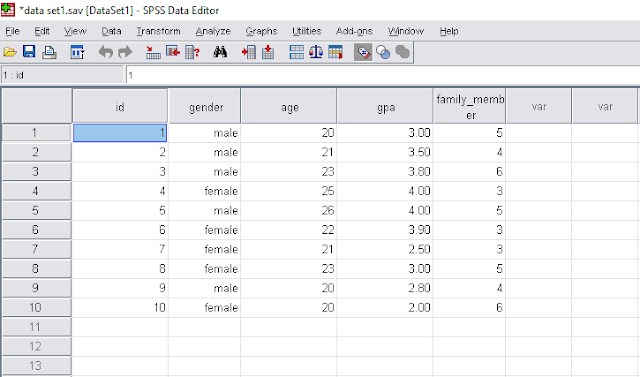Final data set

How to analyze data using SPSS (for beginners)-part 1
How to analyze data using SPSS (part-2), how to input data in SPSS
How to analyze data using SPSS (part-3), how to import data in SPSS from excel file
How to analyze data using SPSS (part-4), how to sort data in SPSS
How to analyze data using SPSS ( part-5) how to merge file in SPSS?
How to analyze data using SPSS ( part-6), how to merge file in SPSS
How to analyze data using SPSS (part-7), Finding missing values, Replacing missing values, Coding missing values in SPSS

How to analyze data using SPSS (part-8), variable transformation, Recoding variables in spss

Univariate analysis| how to analyze data using spss (part-9)
Bivariate analysis| How to analyze data using spss (part-10)